﻿ 改进SURF特征的维吾尔文复杂文档图像匹配检索
«上一篇文章快速检索 高级检索

 智能系统学报2019, Vol. 14Issue (2): 296-305  DOI: 10.11992/tis.2017090140

### 引用本文ALIYA Batur, NURBIYA Yadikar, HORNISA Mamat, et al. Complex Uyghur document image matching and retrieval based on modified SURF feature[J]. CAAI Transactions on Intelligent Systems, 2019, 14(2): 296-305. DOI: 10.11992/tis.201709014.### 文章历史

1. 新疆大学 信息科学与工程学院，新疆 乌鲁木齐，830046;
2. 新疆大学 网络与信息中心，新疆 乌鲁木齐，830046

Complex Uyghur document image matching and retrieval based on modified SURF feature
ALIYA Batur 1, NURBIYA Yadikar 1, HORNISA Mamat 1, ALIMJAN Aysa 2, KURBAN Ubul 11. School of Information Science and Engineering, Xinjiang University, Urumqi 830046, China;
2. Network and information center, Xinjiang University, Xinjiang University, Urumqi 830046, China
Abstract: This study is aimed at the uncertainty and computational complexity of the clustering center in local image features retrieval based on the bag-of-words (BOW) model. A method to retrieve the measure of similarity degree from different kinds of distance and another method that requires using the matching point number as the basis of retrieval are proposed in this paper. In this method, the SURF feature is first modified to effectively reduce feature extraction complexity, and then FLANN (fast library for approximate nearest neighbors) bidirectional matching and KD-Tree + BBF matching are implemented for FAST + SURF features. Feature robustness is verified under different transformation conditions. Finally, all kinds of Uyghur document images that have been classified and sorted based on these two retrieval methods are retrieved. The results of the retrieval experiments indicate that the similarity degree measure retrieval based on distance is inferior to the retrieval based on matching number, and both of these two retrieval strategies can meet the requirements of fast and accurate searching.
Key words: complex document image    Uyghur document image    document image segmentation    feature extraction    SURF feature    FALNN bidirectional matching    KD-Tree+BBF matching    image retrieval

1 FAST+SURF特征提取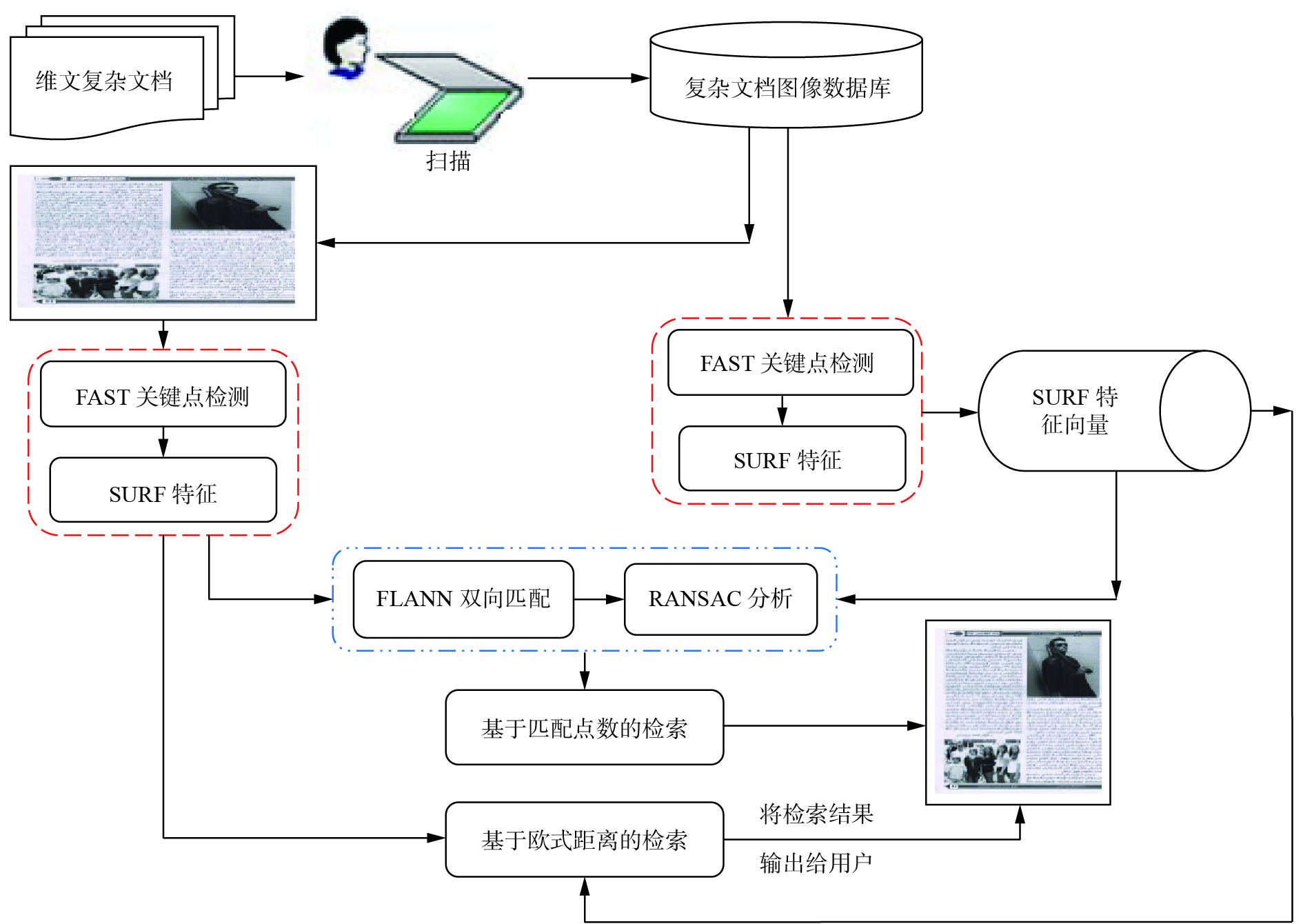Download: 图 1 基于改进SURF特征的维吾尔文印刷体复杂文档图像检索流程框图 Fig. 1 The block diagram of Uyghur printed complex document image retrieval based on modified SURF feature

1.1 原始SURF特征向量提取

 ${I_{{\sum{\left( {X,Y} \right)}}}} = \sum\limits_{i = 0}^{i \ll X} {\sum\limits_{j = 0}^{j \ll Y}} {\rm Gray} \left( {i,j} \right)$ (1)
 $\begin{array}{c} H\left( {x,\sigma } \right) = \left[ {\begin{array}{*{20}{c}} {{L_{xx}}\left( {x,\sigma } \right)} & \;{{L_{xy}}\left( {x,\sigma } \right)}\\ {{L_{yx}}\left( {x,\sigma } \right)} & \;{{L_{yy}}\left( {x,\sigma } \right)} \end{array}} \right]= \\ \left[ {\begin{array}{*{20}{c}} {{D_{xx}}\left( {x,\sigma } \right)} & {{D_{xy}}\left( {x,\sigma } \right)}\\ {{D_{yx}}\left( {x,\sigma } \right)} & {{D_{yy}}\left( {x,\sigma } \right)} \end{array}} \right] \end{array}$ (2)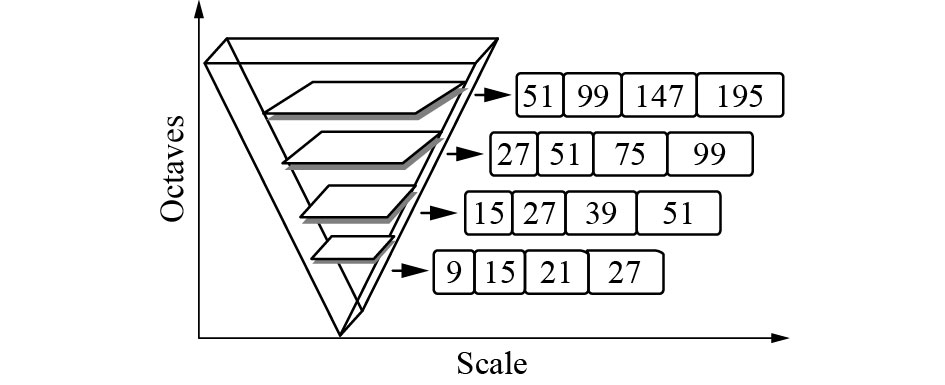Download: 图 2 SURF尺度空间示意 Fig. 2 Schematic diagram of SURF scale space

 ${\rm Det}\left( H \right) = {D_{xx}}{D_{yy}} - {\left( {0.9{D_{{\rm{xy}}}}} \right)^2}$ (3)

1.2 改进SURF特征提取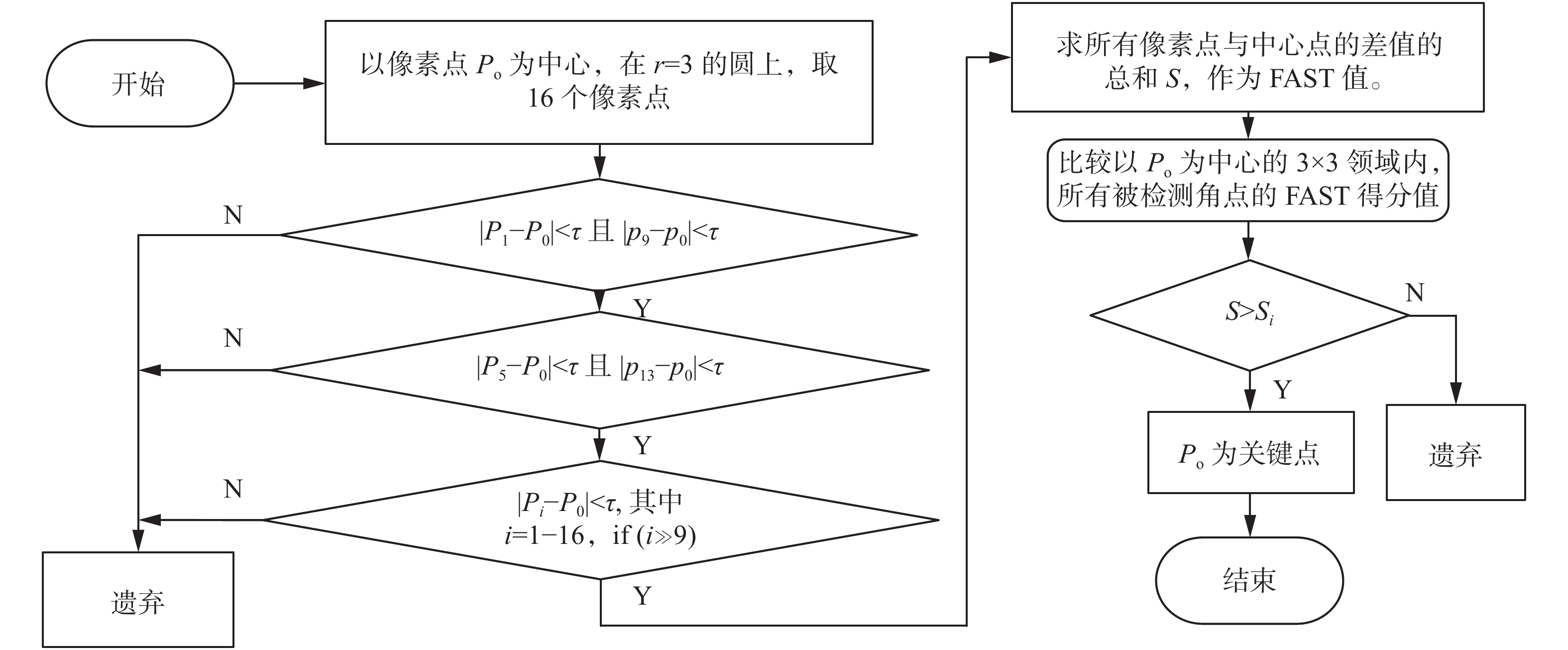Download: 图 3 改进SURF特征关键点检测流程框图 Fig. 3 Flow chart of improved SURF feature key point detection.

2 FAST+SURF特征匹配分析

2.1 双向FLANN匹配Download: 图 4 改进SURF特征双向FLANN匹配示意 Fig. 4 Schematic diagram of improved SURF features bidirectional FLANN matching
2.2 KD−Tree+BBF匹配

KD−Tree的匹配主要由树形结构的建立与最近领查找等两个部分组成。KD−Tree搜索能力与特征向量的维数相关，维数越大，搜索能力越差。因此，本文从改进的KD−Tree出发，将得到的距离结果与预设的阈值相比较，判断是否为匹配关键点。本文中对改进64维SURF特征实现改进KD−Tree匹配的过程如图5所示。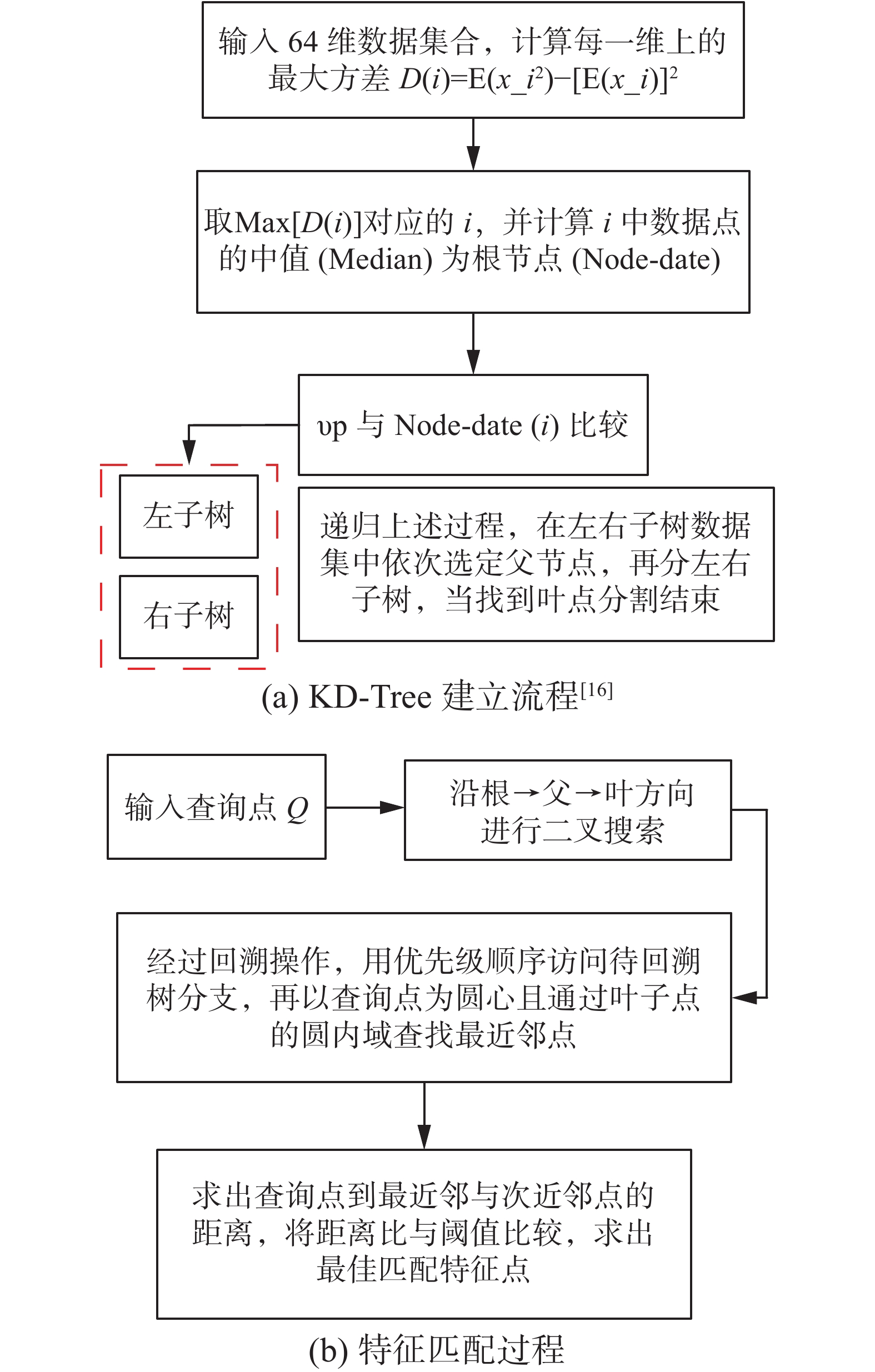Download: 图 5 改进KD−Tree匹配过程描述 Fig. 5 The description of improved KD−Tree matching

 ${\rm MR} =\displaystyle \frac{{{N_c}}}{{{N_d}}}$ (4)
 ${\rm CMR} =\displaystyle \frac{{{N_{ac}}}}{{{N_c}}}$ (5)
 ${\rm IMR} =\displaystyle \frac{{N_{ai}}}{{N_c}}$ (6)

3 维吾尔文复杂文档图像检索

 $d_{\rm{Euclidean}} = \sqrt {{{\left( {{x_1} - {x_2}} \right)}^2} + {{\left( {{y_1} - {y_2}} \right)}^2}}$ (7)
 $d_{\rm{Manhattan}} = \left| {{x_1} - {x_2}} \right| + \left| {{y_1} - {y_2}} \right|$ (8)
 $d_{\rm{Chebyshev}} = \max \left( {\left| {{x_1} - {x_2}} \right|,\left| {{y_1} - {y_2}} \right|} \right)$ (9)
 $d_{\rm{Cosine}} = \frac{{{x_1}{x_{2 + }}{y_1}{y_2}}}{{\sqrt {{x_1}^2 + {x_2}^2} \sqrt {{y_1}^2 + {y_2}^2} }}$ (10)

 $R= \frac{{N - S}}{{N - 1}}$ (11)

4 实验结果与分析 4.1 实验数据

4.2 实验结果与分析

SURF特征在检测的时候主要是靠尺度空间层数(Octaves)、组数(Intervals)、斑点(THRES)阈值的选择。阈值(Octaves，Intervals，Init-sample，THRES)选定的不同，提取的特征点数目也不相同。为验证FAST+SURF特征的效率，在不同的阈值条件下，对1 606×2 290的同一张图像进行实验，统计关键点数和特征提取时间，实验结果如表1给出。表 1 在不同阈值条件下同一幅图像检测的参数统计表 Tab.1 The statistical table of detecting parameters using the same image under different threshold conditions表 2 在同一个阈值下同一幅不同尺寸图像中检测的FAST+SURF关键点与时间对比表 Tab.2 The comparison table of detecting FAST+SURF key points and time using different image sizes in a same threshold表 3 维吾尔文档图像尺寸变换下FAST(100)+SURF特征点的两种匹配结果 Tab.3 Two kinds of FAST (100)+SURF feature points matching results under Uyghur document image scale transform表 4 维吾尔文档图像旋转变换条件下FAST(100)+SURF特征点的两种匹配结果 Tab.4 Two kinds of FAST (100)+SURF feature points matching results under Uyghur document image rotation transform表 5 维吾尔文文档图像光照变换下的FAST(100)+SURF特征点的两种匹配结果 Tab.5 Two types of FAST (100)+SURF feature points matching results with Uyghur document image illumination transform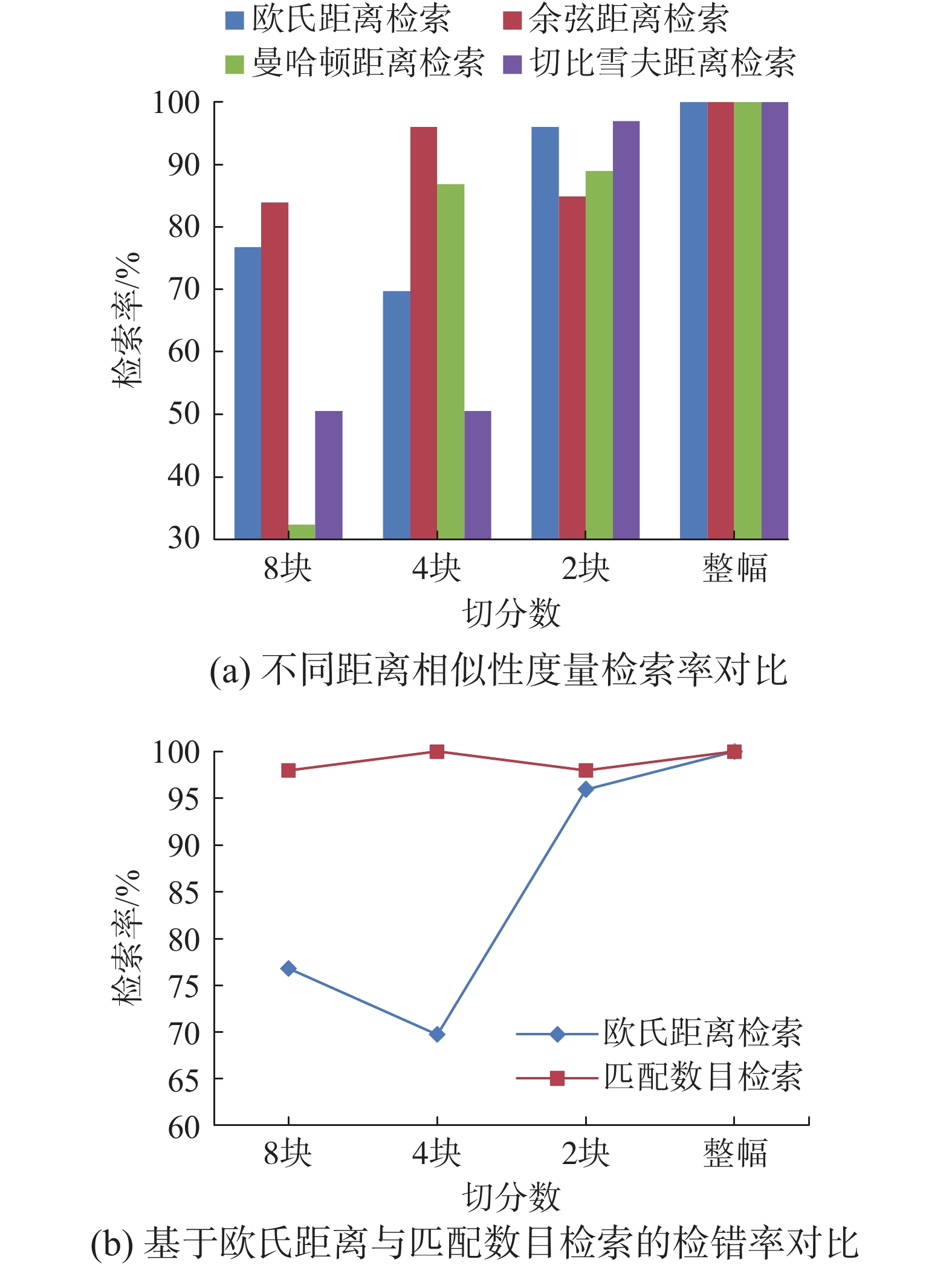Download: 图 6 改进SURF特征实现维吾尔文复杂文档图像检索的性能指标对比 Fig. 6 The performance comparison figure of modified SURF feature based Uyghur complex document image retrieval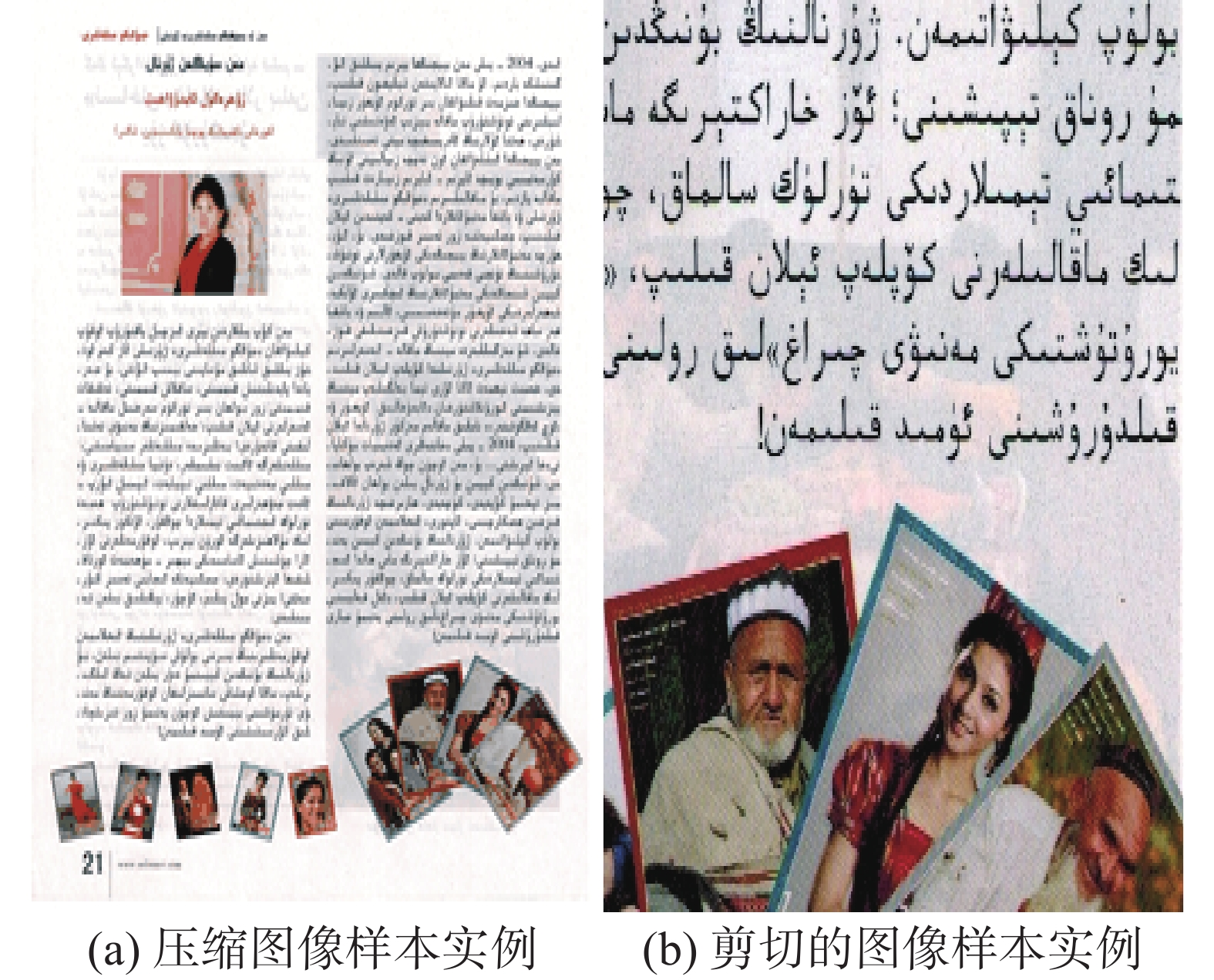Download: 图 7 修改数据库样本实例 Fig. 7 The sample instance of modified database表 8 对剪切文档图像库进行检索实验的统计结果 Tab.8 The statistical results of the sheared Uyghur document image retrieval experiment表 9 对压缩文档图像库进行检索实验的统计结果 Tab.9 The statistical results of the compressed Uyghur document image retrieval experiment

5 结束语

  张敬丽, 张会清, 代汝勇. 基于MIC-SURF的快速图像匹配算法[J]. 计算机工程, 2016, 42(1): 210-214. ZHANG Jingli, ZHANG Huiqing, DAI Ruyong. Fast image matching algorithm based on MIC-SURF[J]. Computer engineering, 2016, 42(1): 210-214. DOI:10.3969/j.issn.1000-3428.2016.01.037 (0)  ALFANINDYA A, HASHIM N, ESWARAN C. Content based image retrieval and classification using speeded-up robust features (SURF) and grouped bag-of-visual-words (GBoVW)[C]//Proceedings of 2013 International Conference on Technology, Informatics, Management, Engineering, and Environment. Bandung, Indonesia, 2013: 77–82. (0)  王澍, 吕学强, 张凯, 等. 基于快速鲁棒特征集合统计特征的图像分类方法[J]. 计算机应用, 2015, 35(1): 224-230. WANG Shu, LYU Xueqiang, ZHANG Kai, et al. Image classification approach based on statistical features of speed up robust feature set[J]. Journal of computer applications, 2015, 35(1): 224-230. (0)  赵璐璐, 耿国华, 李康, 等. 基于SURF和快速近似最近邻搜索的图像匹配算法[J]. 计算机应用研究, 2013, 30(3): 921-923. ZHAO Lulu, GENG Guohua, LI Kang, et al. Images matching algorithm based on SURF and fast approximate nearest neighbor search[J]. Application research of computers, 2013, 30(3): 921-923. DOI:10.3969/j.issn.1001-3695.2013.03.072 (0)  CHEON S H, EOM I K, HA S W, et al. An enhanced SURF algorithm based on new interest point detection procedure and fast computation technique[J]. Journal of real-time image processing, 2016. DOI:10.1007/s11554-016-0614-y (0)  闫利, 陈林. 一种改进的SURF及其在遥感影像匹配中的应用[J]. 武汉大学学报(信息科学版), 2013, 38(7): 770-773, 804. YAN Li, CHEN Lin. A modified SURF descriptor and its application in remote sensing images matching[J]. Geomatics and information science of Wuhan university, 2013, 38(7): 770-773, 804. (0)  陈剑虹, 韩小珍. 结合FAST-SURF和改进k-d树最近邻查找的图像配准[J]. 西安理工大学学报, 2016, 32(2): 213-217, 252. CHEN Jianhong, HAN Xiaozhen. Image matching algorithm combining FAST-SURF and improved k-d tree nearest neighbor search[J]. Journal of Xi’an university of technology, 2016, 32(2): 213-217, 252. (0)  罗楠, 孙权森, 陈强, 等. 结合SURF特征点与DAISY描述符的图像匹配算法[J]. 计算机科学, 2014, 41(11): 286-290, 300. LUO Nan, SUN Quansen, CHEN Qiang, et al. Image matching algorithm combining SURF feature point and DAISY descriptor[J]. Computer science, 2014, 41(11): 286-290, 300. DOI:10.11896/j.issn.1002-137X.2014.11.056 (0)  王亚文, 陈鸿昶, 李邵梅, 等. 基于关键点匹配的多策略尺度自适应跟踪算法[J]. 计算机工程与设计, 2016, 37(1): 247-253. WANG Yawen, CHEN Hongchang, LI Shaomei, et al. Multi-strategy scale adaptive tracking algorithm via keypoint matching[J]. Computer engineering and design, 2016, 37(1): 247-253. (0)  张凤晶, 王志强, 吴迪, 等. 基于SURF的图像配准改进算法[J]. 长春理工大学学报(自然科学版), 2016, 39(1): 112-115. ZHANG Fengjing, WANG Zhiqiang, WU Di, et al. Improved algorithm of image regestration based on SURF[J]. Journal of Changchun university of science and technology (natural science edition), 2016, 39(1): 112-115. DOI:10.3969/j.issn.1672-9870.2016.01.025 (0)  LIU Yanling, MA Sihang. Research on image based on improved SURF feature matching[C]//Proceedings of 7th International Symposium on Computational Intelligence and Design. Hangzhou, China, 2014: 581–584. (0)  EL-GAYAR M M, SOLIMAN H, MEKY N. A comparative study of image low level feature extraction algorithms[J]. Egyptian informatics journal, 2013, 14(2): 175-181. DOI:10.1016/j.eij.2013.06.003 (0)  HUANG Liqin, CHEN Caigan, SHEN Henghua, et al. Adaptive registration algorithm of color images based on SURF[J]. Measurement, 2015, 66: 118-124. DOI:10.1016/j.measurement.2015.01.011 (0)  安维胜, 余让明, 伍玉铃. 基于FAST和SURF的图像配准算法[J]. 计算机工程, 2015, 41(10): 232-235, 239. AN Weisheng, YU Rangming, WU Yuling. Image registration algorithm based on FAST and SURF[J]. Computer engineering, 2015, 41(10): 232-235, 239. DOI:10.3969/j.issn.1000-3428.2015.10.043 (0)  HUI Dong, YUAN Handian. Research of image matching algorithm based on SURF features[C]//Proceedings of 2012 International Conference on Computer Science and Information Processing (CSIP). Xi’an, China, 2012: 1140–1143. (0)  阿丽亚•巴吐尔. 基于局部特征的维吾尔文印刷体复杂文档图像检索研究[D]. 乌鲁木齐: 新疆大学, 2017. ALIYA Batur. Research on Uyghur printed complex document image retrieval based on local feature[D]. Urumchi: Xinjiang University, 2017. (0)